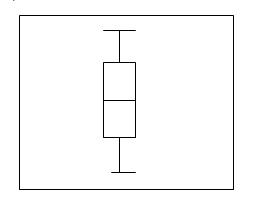# Box Plot

Posted in Statistics, Total Reads: 1892

## Definition: Box Plot

A box plot is a tool for representation of groups of numerical data graphically, through visual representation of quartiles. Quartiles are a ranked set of data values, where 3 prominent points divide the entire data set, into four parts, each part representing quarter of the data. Box plots are generally non parametric, and do not make any prior assumptions.

A box plot can look as follows:Box plots have lines vertical extending from the boxes, which can be referred to as whiskers, it basically indicates variability above and below, outside the quartiles. The distance of space between the different parts of the box indicate the degree of dispersion (spread) and the skewness of the data, Inter quartile, an important term range can be estimated through the box plot.

The major application of a box plot is quick visualization of sets of data together. The advantages of use of box plots over histograms and other diagrams is their relatively smaller space for representation, so comparison of various sets of data becomes easier.

Hence, this concludes the definition of Box Plot along with its overview.

Browse the definition and meaning of more terms similar to Box Plot. The Management Dictionary covers over 7000 business concepts from 6 categories.

Search & Explore : Management Dictionary

Similar Definitions from same Category: